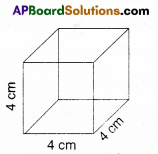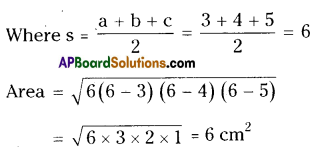# AP Board 9th Class Maths Solutions Chapter 10 Surface Areas and Volumes Ex 10.1

AP State Syllabus AP Board 9th Class Maths Solutions Chapter 10 Surface Areas and Volumes Ex 10.1 Textbook Questions and Answers.

## AP State Syllabus 9th Class Maths Solutions 10th Lesson Surface Areas and Volumes Exercise 10.1

Question 1.
Find the lateral surface area and total surface area of the following right prisms.
i)L.S.A. = 4l2
= 4 × 42
= 64cm2
T.S.A = 6l2
= 6 × 42 = 96cm2ii) L.S.A. =2h(l + b)
= 2 × 5 (8 + 6)
= 10 × 14 = 140 cm2
T.S.A. = 2 (lb + bh + lh)
= 2(8 × 6 + 6 × 5 + 8 × 5)
= 2 (48 + 30 + 40)
= 236 cm2

Question 2.
The total surface area of a cube is 1350 sq.m. Find its volume.
Solution:
Given T.S.A. of a cube 6l2 = 1350
l2 = $$\frac{1350}{6}$$
l2 = 225
∴ l = $$\sqrt{225}$$ = 15m
∴ Volume of the cube = l3
= 15 × 15 × 15
= 3375 m3Question 3.
Find the area of four walls of a room (Assume that there are no doors or windows) if its length 12 m; breadth 10 m and height 7.5 m.
Solution:
Length of the room = 12 m
Breadth of the room = 10 m
Height of the room = 7.5 m
Area of four walls of the room
A = 2h (l + b)
A = 2 × 7.5 (12 + 10)
= 15 × 22
= 330 m2Question 4.
The volume of a cuboid is 1200 cm3. The length is 15 cm and breadth is 10 cm. Find its height.
Solution:
Length of a cuboid, ‘l’ = 15 cm
Breadth of the cuboid, b = 10 cm
Volume of the cuboid, V = lbh = 1200.cm3
Let the height = h
∴ 15 × 10 × h = 1200
∴ h = $$\frac{1200}{15 \times 10}$$
= 8 cmQuestion 5.
How does the total surface area of a box change if
i) Each dimension is doubled ?
Solution:
Let the original dimensions be Length – l units
Height – h units
Then T.S.A = 2 (lb + bh + lh)
If the dimensions are doubled then
Length = 2l
Height = 2h
T.S.A. = 2 (2l. 2b + 2b . 2h + 2l . 2h)
= 2 (4lb + 4bh + 4lh)
= 4 × [2 (lb + bh + lh]
= 4 × original T.S.A.
i.e., T.S.A. increases by 4 times.

ii) Each dimension is tripled ?
Solution:
Let the original and changed dimensions are l, b, h and 31, 3b, 3h
Original T.S.A. = 2 (lb + bh + lh)
Changed T.S.A
= 2 (3l . 3b + 3b . 3h + 3l. 3h)
Changed T S.A. = 2 (9lb + 9bh + 9lh)
= 9 × [2 (lb + bh + lh)]
= 9 [original T.S.A.]
Thus original T.S.A. increased by 9 times if each dimension is tripled.Question 6.
The base of a prism is triangular in shape with sides 3 cm, 4 cm and 5 cm. Find the volume of the prism if its height is 10 cm.
Solution:
Volume of triangular prism = Area of the base × height
Sides of the triangle are 3 cm, 4 cm and 5 cm.
Area = s (s – a) (s – b) (s – c)∴ Volume of the prism = 6 × 10 = 60 cm3
(OR)
3 cm, 4 cm and 5 cm are the sides of right triangle.
∴ Area of the triangle
= $$\frac { 1 }{ 2 }$$ bh = $$\frac { 1 }{ 2 }$$ × 3 × 4 = 6 cm2
Volume of prism = base area × height
= 6 × 10 = 60cm3

Question 7.
A regular square pyramid is 3 m height and the perimeter of its base is16 m Find the volume of the pyramid.
Solution:
Perimeter of the base = 16 m
Height of the pyramid 3 m
Volume of the pyramid
= $$\frac { 1 }{ 3 }$$ × volume of prism
= $$\frac { 1 }{ 3 }$$ × (base area x height)
= $$\frac { 1 }{ 3 }$$ × 4 × 4 × 3= 16m [4 × side=16 ∴ side = 4 m Area = s2 = 4 × 4]Question 8.
An Olympic swimming pool is in the shape of a cuboid of dimensions 50 m long and 25 m wide. If it is 3 m deep throughout, how many litres of water does it hold ?
Solution:
Dimensions of the swimming pool are
Length = 50 m